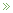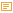• ## Understanding Cross-validation

Posted by Konstantin 17.09.2008

Suppose the other day you came up with a fresh and brilliant idea: to make an automated system that would track the social and economical situation in various countries and predict when and where a new war is going to happen. So you collected historical data on numerous incidents in the world, fed that data into your favourite machine learning model, played around with the parameters, and voilà - you've got something that seems to work. There is just one thing left to do - assess whether the system you created is any good. At the very least, you need to measure its precision: the probability that a positive prediction will in fact be true.

But how do you measure precision in this case? The straightforward way would be to wait a pair of years and compare the numbers of actual and predicted wars on the planet - this would provide an approximate estimate of the true generalization ability of your system. However, this is not a very convenient option, so you better figure out how to assess your new system using only the existing data. And this is when things can get confusing (at least if you think long enough). The most popular solutions here are, of course, holdout testing and cross-validation, but their interpretation is often overlooked, so I believe they are worth thinking about for a minute.

Holdout

Conceptually the simplest way is the holdout validation: you randomly split your data into a training set of size n and a test set of size m, use former for training and estimate performance on the latter. The two things to note here are the following:

• Due to the finite size of the test set you will only get an approximate idea of the true performance. The rule of thumb is that the performance that you measure has an error of the order 1/sqrt(m). You get this number if you try to compute the 99% confidence interval under the normality assumption.
• You are actually measuring the performance of the classifier constructed on your training set, and not the one that you are interested in. This introduces another bias in your measurement. Most often this bias is pessimistic (i.e. the performance of a classifier trained on a smaller set of data is usually worse than the performance of the classifier, trained on the full dataset), however if the dataset contains outliers this need not be the case. I am not aware of any results describing the magnitude of this bias, but I presume that for small datasets it depends on the proportion of data used for training.

Cross-validation

The idea of cross-validation is to repeat the holdout experiment described above k times and average the results.

• If on each iteration your training would result in the same classifier, you could say that the resulting measured performance has an error of the order of 1/sqrt(km). However, each iteration of cross-validation results in a different classifier being built on the selected training set. As a result, you are testing k different classifiers, each with precision 1/sqrt(m), take their average performance, and hope to obtain a better estimate for the performance of your "main" classifier. This hope is justified, because you believe that all of the k classifiers are similar to the "main" one. But what if it is not true? Can it happen so that the variance introduced by the differences in the k classifiers is significant enough to be considered? It is, after all, possible, if the data is very scarce. But again, cross-validation is used precisely when the data is scarce.
• Similarly to the case with holdout, the cross-validation estimate will most often be pessimistically biased, and it seems that no one really knows how large the bias is going to be.

Double Cross-validation

Finally, things get more complicated when you have some "hyper" parameters in your model that you need to tune (such as the λ tradeoff parameter for regularized models). If you simply estimate the performance for a range of parameter values and then pick the one reporting the best value, you are introducing an optimistic bias, because you have in fact tested your algorithm on the same data that was used for training. Therefore, it would be fair to "re-test" your algorithm on some additional data. It is not uncommon to see cases, when a round of cross-validation is used to select the model parameters, and another round is used to estimate the performance (that is, you split the training set in turn into a training and testing sets for model selection). But now things get strange.

• If on each iteration of "outer" cross validation, we re-select the "high-impact" model parameters using "inner" cross-validation, aren't we introducing just too much variability into the k models of the outer round? What performance are we measuring? Is it optimistically or pessimistically biased now? In general, how close should this doubly-cross-validated performance measure to the real model performance? How should this number be interpreted?

If some minutes of thought will help you answer these questions, I believe these would be minutes well worth spending.

Posted by Konstantin @ 8:22 pm

1. Ilja on 17.09.2008 at 22:46 (Reply)

offtopic: next posting on Friday, right?

1. Konstantin on 18.09.2008 at 14:00 (Reply)

This is a very interesting hypothesis that you have come up with! I presume you used past observations to construct a predictive model, and now you are now naturally interested in whether your model predicts correctly.

But how do you measure model precision in this case? The straightforward way would be to wait until Friday and observe, however, this can be somewhat uncomfortable if your patience is weak. So you better figure out how to test your system using only the available data...

Please note: Comment moderation is enabled and may delay your comment. There is no need to resubmit your comment.

### Calendar

September 2008
M T W T F S S
Oct »
1234567
891011121314
15161718192021
22232425262728
2930